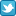Search DSS

Finding Data Analyzing Data Citing data

DSS lab consultation schedule
(Monday-Friday)
 Sep 1-Oct 16 By Zoom appt. here Oct 19-Dec 4 Virtual Zoom Walk-ins Dec 7-Jan 31 By Zoom appt. here Feb 1-April 30 Virtual Zoom Walk-ins May 3-Aug 31 By appt. here
For quick questions email data@princeton.edu.
*No appts. necessary during walk-in hrs.
Note: the DSS lab is open as long as Firestone is open, no appointments necessary to use the lab computers for your own analysis.HomeOnline helpStatistical PackagesS-PlusGraphics

## Graphics

Splus has a powerful graphics environment. Before you can begin to plot things in Splus, you need to open a graphics device. Opening a graphics device is as simple as typing the name of the device and open and close parentheses. Three graphical devices that Splus can use on an X-windows machine include: motif(), X11(), and openlook().

Now type,

• motif()

A new big graphics output window should open. We will only barely scratch the surface of graphics for now, but this list provides a basic introduction to several types.

hist(x)
Makes a frequency histogram of object x. This command takes several subarguments:
boxplot(x)
Creates a boxplot for a vector or for each vector within a data frame.
stem(x)
Creates a stem and leaf plot for a variable. This type of plot is useful for clustering. Note that this does not use the graphics device.
plot(x,y)
Plots variable x against variable y.
abline()
Plots horizontal, vertical, and regression lines in a plot
pairs (object)
Gives the plot for each pair of vectors within a data frame.
brush (object)
Gives all bivariate and multivariate plots for a data frame and allows you to rotate the data and other fun stuff.
identify(x,y)
Interactively identifies points in a plot.

### Plot

The most important of these graphics tools we will deal with today is plot(). Here is the syntax of a basic plotting command (with a couple added perks):

• plot(sd,ud,
xlab= "Social Democratization",
ylab= Ünion Density")

• title("Unionization and Social Democracy")
The xlab and ylab subcommands identify the labels for the x and y axes. The title(titlename) command allows you to input a title for the plot. There are many other options, including varying the color (col) and the the plotting character ( pch). Type ?plot to see your options.

### Adding lines: abline() and lowess() smoothing

You can look graphically represent the fit of your model graphically using the abline() command. This command allows you to plot a fitted line through a plot of points. For example, if you have a model with one dependent and one independent variable, you can use it to look visually at how well the model fits the data.3.

Building on the graph we just made, lets use the abline line command.

• abline(out1)

That's all there is to it. You should note that we can only do this simple function with a bivariate regression. Multiple variables make a single two-dimensional plot impossible.

The second additional line you can add is a smoothed line, one that conforms more exactly to the data than the simple abline(). We'll use a smoother called lowess(). A lowess smoother uses "robust local linear fits" - several regressions, each within a particular window.

To activate this we will need the following commands:

• par(new=T,xaxs="d",yaxs="d")
• plot(lowess(sd,ud),
xlab= "",
ylab= "",
type= "b",
lty=2,
pch=2,
axes=F)
Notice several differences:

par(new=T,xaxs= "d", yaxs= "d")
First we have to tell S-Plus not to delete the current plot when it makes the new one - to overlay the new plot - and to lock in the x and y axes so that we use the same scale.
xlab and ylab
We tell them to be blank so as not to overwrite new text onto our current x and y-axis labels.
type= "b"
This tells S-Plus to plot a line, as well as points
lty=2
This uses a different line type from the abline command to make it easier to distinguish the two lines.
pch=2
Similarly, we can change the plotting character to distinguish these points from the points in the first plot.
axes=F
This is not as important because the two plots are on the same scale, but if they were not (if we were using two x-axes) we would need it.